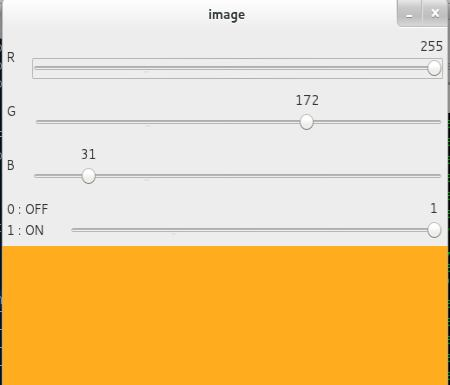# 轨迹栏作为调色板

### 目标

• 了解将轨迹栏固定到OpenCV窗口
• 您将学习以下功能：cv.getTrackbarPoscv.createTrackbar等。

### 代码演示

``````import numpy as np
import cv2 as cv
def nothing(x):
pass
# 创建一个黑色的图像，一个窗口
img = np.zeros((300,512,3), np.uint8)
cv.namedWindow('image')
# 创建颜色变化的轨迹栏
cv.createTrackbar('R','image',0,255,nothing)
cv.createTrackbar('G','image',0,255,nothing)
cv.createTrackbar('B','image',0,255,nothing)
# 为 ON/OFF 功能创建开关
switch = '0 : OFF \n1 : ON'
cv.createTrackbar(switch, 'image',0,1,nothing)
while(1):
cv.imshow('image',img)
k = cv.waitKey(1) & 0xFF
if k == 27:
break
# 得到四条轨迹的当前位置
r = cv.getTrackbarPos('R','image')
g = cv.getTrackbarPos('G','image')
b = cv.getTrackbarPos('B','image')
s = cv.getTrackbarPos(switch,'image')
if s == 0:
img[:] = 0
else:
img[:] = [b,g,r]
cv.destroyAllWindows()
``````### 练习题

1. 使用轨迹栏创建颜色和画笔半径可调的Paint应用程序。有关绘制的信息，请参阅有关鼠标处理的先前教程。